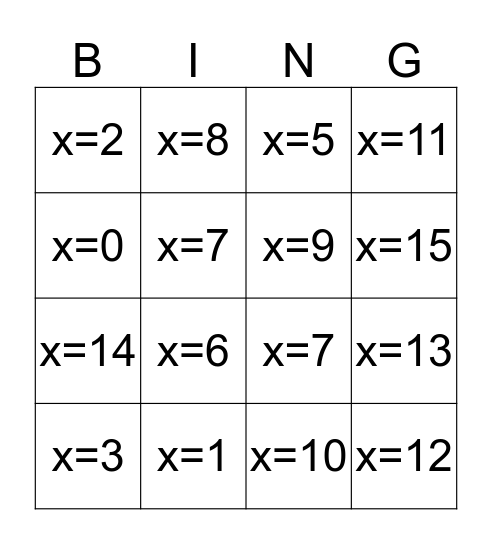# Untitled BingoThis bingo card has 16 words: x=3, x=1, x=5, x=11, x=0, x=8, x=7, x=13, x=2, x=7, x=10, x=15, x=14, x=6, x=9 and x=12.

⚠ This card has duplicate items: x=7 (2)

## Play Online